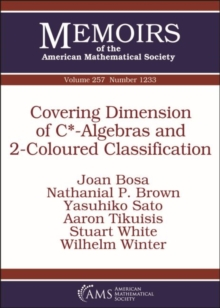Supporting your high street Find out how »
• My Account# Covering Dimension of C*-Algebras and 2-Coloured Classification Paperback / softback

## Part of the Memoirs of the American Mathematical Society series

#### Description

The authors introduce the concept of finitely coloured equivalence for unital $^*$-homomorphisms between $\mathrm C^*$-algebras, for which unitary equivalence is the $1$-coloured case.

They use this notion to classify $^*$-homomorphisms from separable, unital, nuclear $\mathrm C^*$-algebras into ultrapowers of simple, unital, nuclear, $\mathcal Z$-stable $\mathrm C^*$-algebras with compact extremal trace space up to $2$-coloured equivalence by their behaviour on traces; this is based on a $1$-coloured classification theorem for certain order zero maps, also in terms of tracial data. As an application the authors calculate the nuclear dimension of non-AF, simple, separable, unital, nuclear, $\mathcal Z$-stable $\mathrm C^*$-algebras with compact extremal trace space: it is 1.

In the case that the extremal trace space also has finite topological covering dimension, this confirms the remaining open implication of the Toms-Winter conjecture.

Inspired by homotopy-rigidity theorems in geometry and topology, the authors derive a homotopy equivalence implies isomorphism'' result for large classes of $\mathrm C^*$-algebras with finite nuclear dimension.

#### Information

• Format: Paperback / softback
• Pages: 97 pages
• Publisher: American Mathematical Society
• Publication Date:
• Category: Calculus & mathematical analysis
• ISBN: 9781470434700

£84.50

£83.65We're closed for now, but hopefully not for long…

You can still add items to your basket and wishlist but you won't be able to checkout.

£88.50

£87.55

£88.50

£87.55

£88.50

£87.55

£88.50

£87.55